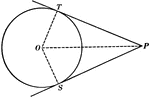### Equal Tangents to Circle Theorem

Illustration used to show that "If two tangents are drawn from any given point to a circle, those tangents…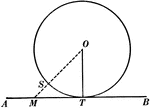### Tangent to Perpendicular Radius Circle Theorem

Illustration used to show that "A tangent to a circle is perpendicular to the radius drawn to the point…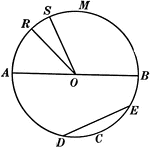### Chords, Diameters, and Radii of a Circle

Illustration of a circle with diameter AB. Radii are RO, SO, AO, and BO. ED is a chord.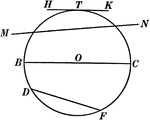### Chords, Secants, Diameters, and Tangents of a Circle

Illustration of a circle with diameter BC, chord DF, secant MN, and tangent HK.### Quadrilateral Inscribed In A Circle

An illustration showing a quadrilateral inscribed in a circle that is tangent to a line.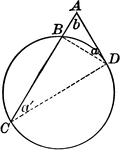### Circle With Tangent and Secant

Circle with secant and tangent drawn.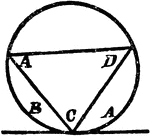### Triangle Inscribed In A Circle

An illustration showing a triangle with angles A, C, and D inscribed in a circle which is tangent to…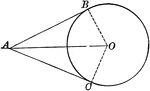### Circle With Two Tangents Drawn From an External Point

Illustration of a circle which illustrates that the tangents to a circle drawn from an external point…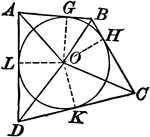### Circle With Various Chords And Tangents

Illustration showing a circle with various chords and tangents drawn.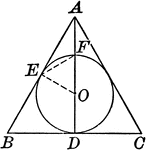### Circle With Various Chords And Tangents

Illustration showing a circle with various chords and tangents drawn.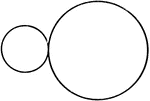### 2 Externally Tangent Circles

Illustration of two circles that are externally tangent to each other.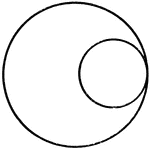### 2 Internally Tangent Circles

Illustration of two circles that are internally tangent to each other.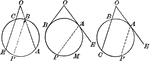### Circles With Angles Formed by Secants and Tangents

Illustration showing angles formed by two secants, two tangents, or a tangent and a secant, drawn to…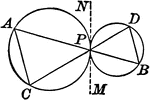### Tangent Circles With Chords

Illustration showing 2 circles with that touch each other and two lines drawn through the point of contact…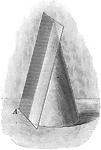### Tangent Plane to a Cone

Illustration of a plane tangent to a cone which contains one element of the cone but does not cut the…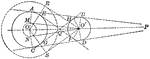### Construction of a Common Tangent When Given Two Circles

Illustration used to construct a common tangent when given two circles.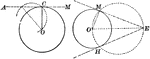### Construction of Tangent Line Through a Given Point to a Given Circle

Illustration of the construction used to draw a tangent to a given circle through a given point.### Parallel Tangents to an Ellipse

Illustration showing that tangents drawn at the ends of any diameter are parallel to each other.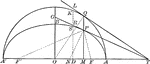### Tangents to an Ellipse

Illustration showing the tangents drawn at two corresponding points of an ellipse and its auxiliary…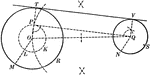### Construction of an External Tangent to 2 Circles

Illustration used to show how "to construct a common external tangent to two given circles."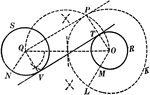### Construction of an 2 External Tangents to 2 Circles

Illustration used to show how to construct two common external tangents to two given circles.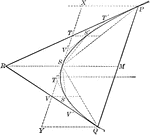### Tangents and Chords of a Parabola

Illustration showing that the area of a parabolic segment made by a chord is two thirds the area of…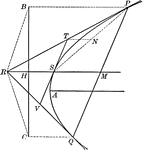### Tangents to a Parabola

Illustration showing that if two tangents RP and RQ are drawn from a point R to a parabola, the line…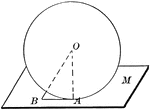### Sphere Tangent to Plane

Diagram used to prove the theorem: "A plane perpendicular to a radius at its extremity is tangent to…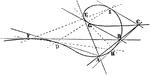### Satellite

"Nodal Cubic, with four primary lines and their satellite. In the diagram, ABC is the satellite line.…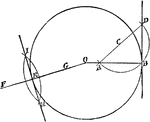### Construction of Tangent to a Circle

Illustration used to draw a tangent to a circle.### Construction of a Tangent to a Circle

Illustration used to show how "to construct a tangent to a circle from a point outside."